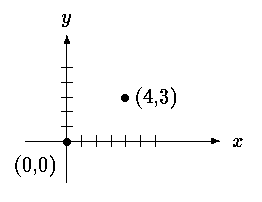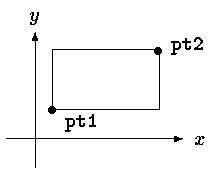# Programming Tutorials

## Basics of Structures in C

By: Grenfel Printer Friendly Format

The keyword struct introduces a structure declaration, which is a list of declarations enclosed in braces. An optional name called a structure tag may follow the word struct (as with point here). The tag names this kind of structure, and can be used subsequently as a shorthand for the part of the declaration in braces.

The variables named in a structure are called members. A structure member or tag and an ordinary (i.e., non-member) variable can have the same name without conflict, since they can always be distinguished by context. Furthermore, the same member names may occur in different structures, although as a matter of style one would normally use the same names only for closely related objects.

Let us create a few structures suitable for graphics. The basic object is a point, which we will assume has an x coordinate and a y coordinate, both integers.The two components can be placed in a structure declared like this:

```   struct point {
int x;
int y;
};
```

A struct declaration defines a type. The right brace that terminates the list of members may be followed by a list of variables, just as for any basic type. That is,

```   struct { ... } x, y, z;
```
is syntactically analogous to
```   int x, y, z;
```
in the sense that each statement declares x, y and z to be variables of the named type and causes space to be set aside for them.

A structure declaration that is not followed by a list of variables reserves no storage; it merely describes a template or shape of a structure. If the declaration is tagged, however, the tag can be used later in definitions of instances of the structure. For example, given the declaration of point above,

```   struct point pt;
```
defines a variable pt which is a structure of type struct point. A structure can be initialized by following its definition with a list of initializers, each a constant expression, for the members:
```   struct maxpt = { 320, 200 };
```
An automatic structure may also be initialized by assignment or by calling a function that returns a structure of the right type.

A member of a particular structure is referred to in an expression by a construction of the form

structure-name.member

The structure member operator ``.'' connects the structure name and the member name. To print the coordinates of the point pt, for instance,

```   printf("%d,%d", pt.x, pt.y);
```
or to compute the distance from the origin (0,0) to pt,
```   double dist, sqrt(double);

dist = sqrt((double)pt.x * pt.x + (double)pt.y * pt.y);
```
Structures can be nested. One representation of a rectangle is a pair of points that denote the diagonally opposite corners:```   struct rect {
struct point pt1;
struct point pt2;
};
```
The rect structure contains two point structures. If we declare screen as
```   struct rect screen;
```
then
```   screen.pt1.x
```
refers to the x coordinate of the pt1 member of screen.

 Most Viewed Articles (in C ) Latest Articles (in C)

Comment on this tutorial